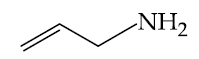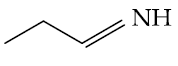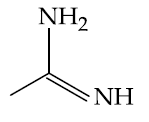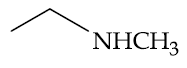# The increasing order of basicity of the following compounds is:(a)(b)(c)(d)Option 1) (d) < (b) < (a) < (c) Option 2) (a) < (b) < (c) < (d) Option 3) (b) < (a) < (c) < (d) Option 4) (b) < (a) < (d) < (c)

A Anuj Pandey
S subam

As we learned

Comparision of Basic nature in aliphatic amines in gaseous phase -

The +I effect of the alkyl group, which increase the electron density on N atom, facilitates the nitrogen atom to use its unshared pair of electrons for bond formation. Thus R - NH2 is expected to be more basic than NH3. Going by the same arguments the basic strength of amines is expected to increase with the increase in the number of alkyl groups attached to the nitrogen atom.

- wherein

(b) –lone pair present on  hybridized nitrogen

(a)  of  hybridized carbon

(d)  of two alkyl group

(c) Guanidine derivative

Option 1)

(d) < (b) < (a) < (c)

Option 2)

(a) < (b) < (c) < (d)

Option 3)

(b) < (a) < (c) < (d)

Option 4)

(b) < (a) < (d) < (c)

Exams
Articles
Questions